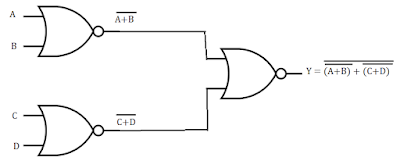# REALIZATION OF BOOLEAN EXPRESSIONS AND LOGIC FUNCTIONS USING ONLY NOR GATES -

### Example-1. Implement Boolean expression Y = (A+B).(C+D) using only NOR gates.

Solution: Y = (A+B).(C+D)

Step-1: Take double bar on both sides of the above expression

Y// = [(A+B).(C+D)]//

Step-2: Apply De Morgan’s Theorem to the inner bar of the right side of the above expression

Y// = Y = [(A+B)/+(C+D)/]/

Step-3: The above expression can easily be implemented using NOR GATES as shown in Fig. 1:Fig. 1

### Example-2. Implement F(A, B, C) = ΠM (0, 1, 2, 4) using NOR gates

Solution: To design a logic circuit using NOR gates, its logic function is to be written in POS (Product of Sum) form.

Using K-Map the logic function F(A, B, C) = ΠM (0, 1, 2, 4)  is simplified and written in SOP form as given below:

K-Map:

Boolean Expression of the given logic function in POS form will be

F(A, B, C) = (A+B).(B+C).(C+A)

Now, follow all the three steps of Example-1. The circuit would be:

Hope this post on "REALIZATION OF BOOLEAN EXPRESSIONS AND LOGIC FUNCTIONS USING ONLY NOR GATES" would be helpful to gain knowledge on how to implement any digital circuit using NOR Gates only.